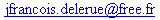My homepage; DXScm; 3D fractals ;

At the age of 8, in 1981, I made my first discovery of a Lisp like languages with the Logo. It was on a Texas Instrument TI-99/4A . Because of the small size of the random memory, the only way to draw on the screen was to redefine some of the 255 characters. And because lot of them had to be kept to display messages, only a few of them were used by the turtle to draw her path. The result was a frustrating "no more ink" after a few lines drawn on the screen.

The aim of this small program is to implement a 3D turtle in Scheme using OpenDX and DXScm. I do not try to redine the whole LOGO language into scheme (for a full LOGO, see WinLogo), but only the few command necessary to command the turtle:

 forward (forward X) Move forward X steps. backward (backward X) Move backward X steps. right (right X) Turn on the right by X degrees. left (left X) Turn on the left by X degrees. dive (dive X) Turn down by X degrees. up (up X) Turn up by X degrees. roll-right (roll-right X) Roll on the right by X degrees. roll-left (roll-left X) Roll on the left by X degrees. pen-up (pen-up ) Stop to draw the path of the turtle. pen-down (pen-down ) Start again to draw the path of the turtle. pen-color (pen-color C) Set the color of the next path to C. It should be a list of three numbers between 0 and 1, representing the percent of red, green and blue. triangle (triangle C) Draw a triangle using the last three point defined by the rotation of the turtle. C is optional and represent the color of the face. It should be a list of three numbers between 0 and 1, representing the percent of red, green and blue. triangle-color (triangle-color C) Set the color of the next triangles to C. It should be a list of three numbers between 0 and 1, representing the percent of red, green and blue. repeat (repeat N A) This function does not directly control the turtle but is much simpler than the "do" structure in Scheme and can be used instead. N is a positive integer, A a command or a list of command. This will repeat N times the command A.

A description of the implementation can be found here.

Here are some small examples:

1. A square!

This first program ask the turtle to repeat 4 times the command: go forward 30 units and turn right 90 degrees.

```(define (program1 )

(repeat 4
(forward 30)
(right 90)
)
)```

2. An house!

This second program combine different distance and angles to draw a pretty house (tips: a compass is sometime necessary for masterpiece):

```(define (program2 )
(forward 40)
(right 52.5)
(forward 50)
(right 75)
(forward 50)
(right 52.5)
(forward 40)
(right 90)
(forward 10)
(right 90)
(forward 30)
(left 90)
(forward 20)
(left 90)
(forward 30)
(right 90)
(forward 50)
)```
`To add a roof to that house, it is possible to add a (triangle '(0.99 0.0 0.0)) just after the second (right 52.5).`

3. A cube!

This is more complex! A first example shows how to draw the cube face after face and a second one use a "do" loop.

3.1

```(define (program31 )
(repeat 4 (forward 30) (right 90))
(repeat 4 (forward 30) (right 90))
(repeat 4 (forward 30) (right 90))
(repeat 4 (forward 30) (right 90))
(repeat 4 (forward 30) (right 90))
(repeat 4 (forward 30) (right 90))
)```

3.2

```(define (program32 )
)```last update: 3/06/03